# Analysis of the Impacts of Open Residential Communities on Road Traffic Based on AHP and Fuzzy Theory

Analysis of the Impacts of Open Residential Communities on Road Traffic Based on AHP and Fuzzy Theory
Xi'an International University, Xi'an 710077, China

Corresponding Author Email:
Page:
183-190
|
DOI:
https://doi.org/10.18280/isi.250205
1 December 2019
|
Accepted:
18 February 2020
|
Published:
15 May 2020
| Citation

OPEN ACCESS

Abstract:

Keywords:

open residential community, AHP, fuzzy comprehensive evaluation, VISSIM traffic simulation

1. Introduction

This paper first establishes an evaluation indicator system from three aspects - road capacity, road safety and road vulnerability. Through multiple screenings, this paper decides to select 5 indicators, namely traffic density, traffic delay time, number of intersection conflicts, road congestion rate and road accessibility to reflect the surrounding road traffic conditions before and after a residential community is opened. Then, based on these indicators, this paper establishes an analytic hierarchy structure model, calculates the weights of these indicators, and passes the consistency check to make sure the reliability of the indicator system. After that, based on the analytic hierarchy model, it establishes a fuzzy comprehensive evaluation model and performs quantitative evaluation on road traffic. At last, based on the above evaluation indicator system and model and by applying the VISSIM traffic simulation technique, this paper performs a simulation test on a residential community and compares and analyze the test results through the grey relational algorithm.

2. Road Traffic Evaluation Indicator System

This paper first establishes an evaluation indicator system from three aspects - road capacity, road safety and road vulnerability [16, 17]. After multiple screenings, this paper decides to use five indicators, namely traffic density, traffic delay time, number of intersection conflicts, road congestion rate and road accessibility to reflect the surrounding road traffic conditions before and after the opening of a residential community.

2.1 Description of evaluation indicators

2.1.1 Traffic density

Traffic density refers to the number of vehicles distributed on a unit length of a lane at a certain instant of time, which reflects the concentration degree of vehicle distribution. The calculation formula is as follows:

$\text{K}=\text{N/L (pcu/km) }$               (1)

where, K is the traffic density; N is the number of vehicles on a single-lane road segment (pcu); L is the length of the road segment.

2.1.2 Traffic delay time

The traffic delay time at intersections is one of the important indicators to measure the efficiency of traffic operations. The opening of a residential community may affect the traffic delay time at intersections . Through comparison of the delay time before and after community opening, the impact on road traffic capacity can be obtained.

The calculation formula is as follows:

$\text{W}=\frac{(\rho +\frac{{{\rho }^{2}}+q_{0}^{2}\frac{1}{{{q}^{2}}}}{2(1-\rho )})}{q}$           (2)

where, W is the traffic delay time at the intersection; ρ is the utilization rate (service intensity) of the entrance, which is the ratio of the average number of vehicles arriving at the intersection to the average number of vehicles that can be serviced at the same time, that is, the probability of any vehicle waiting on the stop line at the intersection; q is the average traffic flow at the intersection; and q0 is the specific traffic flow at a certain intersection.

2.1.3 Number of intersection conflicts

The number of intersection conflicts (this paper only studies the situations at intersections with no traffic light) can be used as a safety evaluation indicator . The theoretical formula to estimate the number of conflicts per hour at a road intersection is as follows:

$\text{N}=3600{{H}_{0}}({{\lambda }_{1}}{{e}^{-{{\lambda }_{1}}{{H}_{0}}}})({{\lambda }_{2}}{{e}^{-{{\lambda }_{2}}{{H}_{0}}}})$             (3)

where, N is the number of intersection conflicts (theoretical estimate); and $\lambda_{1}$ and $\lambda_{2}$ are the traffic flow on the trunk road and the branch road (residential community road), respectively; and H0 is the threshold of the time interval between the arrivals of vehicles from the residential community road and the trunk road at the statistical cross section.

Road congestion rate is the ratio of the actual traffic volume on a road segment to the evaluation benchmark for 24 hours a day or 12 hours during the daytime .

The calculation formula is as follows:

$\text{D}C=\frac{\varpi *\text{Q}}{C}$          (4)

where, $D C$ is the congestion rate; $\varpi$ is the weight coefficient, and $\varpi=1-\alpha / 100+\alpha * \beta / 100 ; \alpha$ is the mixing rate of oversize vehicles; Q is the total traffic volume during the 12-hour daytime; and C is the evaluations benchmark for 12-hour traffic volume.

Road accessibility refers to the relative difficulty of moving from any point to another point in the road network, and factors related to this indicator include distance, time, cost and so on. Road accessibility in fact reflects the road resistance to a certain horizontal movement process . The overall accessibility of an urban road is expressed as weighted accessibility . It can be calculated as the weighted average of the accessibility of each OD point pair with the traffic load as the weight, that is,

$\gamma =\frac{1}{\sum\limits_{\text{A},B\in l}{{{U}_{AB}}}}\sum\limits_{A,B\in l}{{{U}_{AB}}{{\gamma }_{AB}}}$                (5)

where, $U_{A B}$ is the traffic volume of $A \rightarrow B ; \gamma_{A B}$ is the accessibility of $A \rightarrow B$. With the residential community accessibility as an example, the weighted accessibility indicator can be calculated as:

$\gamma =\frac{1}{\sum\limits_{\text{i}=1}^{I}{\sum\limits_{j=1}^{J}{{{U}_{ij}}}}}\sum\limits_{i=1}^{I}{\sum\limits_{j=1}^{J}{{{U}_{ij}}{{\gamma }_{ij}}}}$             (6)

For ease of expression and calculation, it can be defined that when i=j, $U_{i j}=0, \gamma_{i j}=1$.

2.2 AHP modelling

2.2.1 Establishment of the hierarchy structure

The Analytic Hierarchy Process (AHP) is to decompose the decision problem into different hierarchical levels in the order of overall objectives, sub-objectives at each layer, evaluation criteria and specific schemes, then calculate the priority value of each element on a layer to a certain element on the immediate upper layer by solving the feature vector of the judgment matrix, and at last recursively obtain the final weight of each scheme to the overall objective by the weighted sum method . The one with the largest final weight is the optimal solution.

To apply the AHP in the comprehensive evaluation of the impacts of an open residential community on road traffic, a hierarchical analysis model must be established first. Through analysis, it can be known that the indicators affecting road traffic are logically related in a way that sub-class indicators reflect a main class indicator while main class indicators reflect the road traffic capacity. This logical relationship is hierarchical, decomposing elements related to decision-making into objective, criteria and schemes, as shown in Figure 1.

## 1.png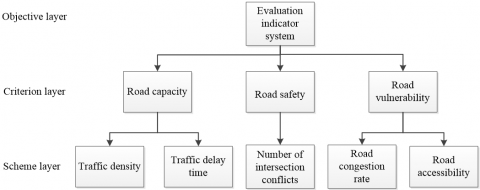Figure 1. Hierarchy structure

2.2.2 Weight analysis of evaluation indicators based on AHP

The weight of each influencing factor in the criterion layer is determined, forming a pairwise comparison matrix as shown below:

$\text{M}=\left( \begin{matrix} 1 & 3/4 & 4/3 \\ 4/3 & 1 & 3/2 \\ 3/4 & 2/3 & 1 \\\end{matrix} \right)$.

Through calculation with the software MATLAB, the feature vector corresponding to the maximum feature root is:

$\beta=(0.3280 .4130 .260)$.

Consistency check is carried out to determine whether the result is feasible. Through calculation, there is:

$CI=\frac{\lambda \max -n}{n-1}=0$.

The values of the random consistency index RI are shown in Table 1.

Table 1. Values of the consistency index RI

 n 1 2 3 4 5 6 7 8 RI 0 0 0 0.9 1.12 1.24 1.32 1.41
At this time: CR=CI/RI=0/0.90=0<0.1.

It shows that the results are feasible. The weights of the influencing factors in the criterion layer are shown in Table 2.

Table 2. Weights of the influencing factors in the criterion layer

 Indicator Weight Consistency check Traffic capacity A $\varpi 1$=0.328 $\Sigma \varpi_{i}=1 \quad \lambda \max =3$ CI=0 CR=0<0.1 Safety B $\varpi 2$=0.413 Vulnerability C $\varpi 3=0.260 Similarly, the weight of each influencing factor in the scheme layer under the impacts of traffic capacity, safety and vulnerability can be calculated. The weight of traffic density is: ω1=0.178*0.328+0.092*0.413+0.212*0.260=0.152. The weight of each influencing factor in the scheme layer is shown in Table 3. Table 3. Weight of each influencing factor in the scheme layer  Indicator Weight Traffic density ω1=0.159 Traffic delay time ω2=0.123 Traffic saturation ω3=0.115 Number of intersection conflicts ω4=0.127 Road congestion rate ω5=0.471 Through the above analysis, it can be seen that, in the order of weight, the influencing factors in the scheme layer are as follows: Road congestion rate, traffic density, number of intersection conflicts, traffic delay time and traffic saturation. 2.3 Establishment of the fuzzy comprehensive evaluation model 2.3.1 Overview of the fuzzy comprehensive evaluation model The fuzzy comprehensive evaluation method is a comprehensive evaluation method based on fuzzy mathematics. It converts qualitative evaluation to quantitative evaluation according to the membership theory of fuzzy mathematics; in other words, it uses fuzzy mathematics to make an overall evaluation of the things or objects restricted by multiple factors. With clear results and strong systematicness, this method can solve problems that are fuzzy and difficult to quantify, and thus is suitable for solving various uncertainty problems. The concept of fuzzy set theory was proposed in 1965 by Professor Chad, the American automatic control expert, to express the uncertainty of things . 2.3.2 Basic steps of the fuzzy comprehensive evaluation model (1) Determine the factor set Road traffic needs to be comprehensively evaluated from multiple aspects. This paper directly uses the evaluation indicator system previously established as the factor set, which is denoted as: U={u1, u2, ..., un}, where, ui (i=1, 2, ..., n) represents each influencing factor. (2) Determine the remark set Due to the different evaluation results of the indicators, there will be different ratings. For example, for work performance, the ratings include “excellent”, “good”, “medium”, “poor” and “very poor”. The set consisting of different evaluation results is called a remark set, denoted as: V={v1, v2, ..., vm}, where, vi (i=1, 2, ..., m) represents each rating. (3) Determine the weight of each factor In general, different factors in the factor set have different contributions in the comprehensive evaluation. The comprehensive evaluation results are not only related to the evaluation result of each factor, but also dependent to a large extent on how these factors contribute to the comprehensive evaluation. This indicates that the weight distribution of all these factors has to be determined. It is a fuzzy vector on U, denoted as: A={a1, a2, …, an}, where, ai represents the weight of the i-th factor, which satisfies$\sum_{i=1}^{n} a_{i}=1$. There are many methods for determining the weights, such as the Delphi method, the weighted average method, and the crowd evaluation method etc. (4) Determine the fuzzy comprehensive judgment matrix For the indicator ui, each remark is a fuzzy subset on V. The evaluation of the indicator ui is denoted as: Ri={Ri1, Ri2, ..., Rim}, The fuzzy comprehensive judgment matrix of each indicator is:$R=\left[ \begin{matrix}   {{r}_{11}} & {{r}_{12}} & ... & {{r}_{1m}}  \\   {{r}_{21}} & {{r}_{22}} & ... & {{r}_{2m}}  \\   ... & ... & ... & ...  \\   {{r}_{n1}} & {{r}_{n2}} & ... & {{r}_{nm}}  \\\end{matrix} \right]$. It is a fuzzy relation matrix from U to V. (5) Perform comprehensive evaluation If there is a fuzzy relationship from U to V, which is R=(Rij)n×m, then a fuzzy transformation $T_{R}: F(U) \rightarrow F(V)$can be obtained using R, and from this transformation, the comprehensive evaluation result can be obtained, which is B=A•R. The comprehensive evaluation can be regarded as a fuzzy vector on V, denoted as B={b1, b2, ..., bm}. 2.3.3 Application of the fuzzy comprehensive evaluation model in this study (1) Set the factor set as: U={traffic density u1, traffic delay time u2, number of intersection conflicts u3, road congestion rate u4, road accessibility u5}. (2) Set the remark set as: V={good v1, relatively good v2, average v3, relatively poor v4, poor v5}. (3) Determine the weight of each factor Based on the weight of each indicator obtained, list the weight vector A={0.159, 0.123, 0.115, 0.127, 0.471}. (4) Determine the fuzzy comprehensive evaluation matrix, and rate each ui. For example, u1 is determined based on the score given by the expert panel. Suppose the scores are as follows: R1=[0.1, 0.2, 0.2, 0.3, 0.2]. The above expression indicates that 10% of the experts participating in the scoring think that the traffic density is good, 50% think that the traffic density is relatively good, 40% think that the traffic density is average, and 0 expert think that the traffic density is relatively poor or poor. The other factors are also evaluated in the same way. u2, u3, u4 and u5 are also scored by the expert panel: R2=[0.2, 0.3, 0.5, 0, 0], R3=[0.2, 0.2, 0.4, 0.1, 0.1], R4=[0.1, 0.1, 0.5, 0.3, 0] and R5=[0.2, 0.2, 0.2, 0.2, 0.2]. Let Ri be line i to form the evaluation matrix:$R=\left[ \begin{matrix}   0.1 & 0.2 & 0.2 & 0.3 & 0.2  \\   0.2 & 0.3 & 0.5 & 0 & 0  \\   0.2 & 0.2 & 0.4 & 0.1 & 0.1  \\   0.1 & 0.1 & 0.5 & 0.3 & 0  \\   0.2 & 0.2 & 0.2 & 0.2 & 0.2  \\\end{matrix} \right]$. It is a fuzzy relation matrix from the factor set U to the remark set V. (5) Fuzzy comprehensive evaluation Perform compositional operation of the matrix. B=A•R=[0.159, 0.123, 0.115, 0.127, 0.471]$\left[ \begin{matrix}   0.1 & 0.2 & 0.2 & 0.3 & 0.2  \\   0.2 & 0.3 & 0.5 & 0 & 0  \\   0.2 & 0.2 & 0.4 & 0.1 & 0.1  \\   0.1 & 0.1 & 0.5 & 0.3 & 0  \\   0.2 & 0.2 & 0.2 & 0.2 & 0.2  \\\end{matrix} \right]$=[0.1704, 0.1986, 0.2970, 0.1915, 0.1373] According to Table 4, the remark with the greatest value is taken as the comprehensive evaluation result, which is “average”. Table 4. Evaluation results and ratings  Poor Relatively poor Average Relative good Good <0.1 [0.1, 0.2) [0.2, 0.35) [0.35, 0.5) ≥0.5 3. Effect Analysis of Open Residential Communities 3.1 Definitions of open residential community types In most of the research on open residential communities, the internal road structure of the residential community was used as the sole criterion for the classification of residential communities. This paper categorizes residential communities according to the different effects on road traffic. The internal road structure of the residential community is only one of the factors affecting traffic, and not the main factor. The area of the residential community and the traffic volume around the residential community should be treated as the main criteria for the classification of residential communities. The block-type residential community is the most common form adopted in current residential area planning. This study further divides the block-type residential communities. With area and surrounding traffic volume as the criteria, residential communities are classified into 4 types, namely large area and large traffic volume, large area and small traffic volume, small area and large traffic volume, and small area and small traffic volume (referred to as Type A, Type B, Type C and Type D residential communities respectively in this paper). Definitions of these types of residential communities are listed in Table 5. Table 5. Definitions of different types of residential communities  Type A residential community Area of 25-35 ha. (375-525 mu) and high traffic volume Type B residential community Area of 25-35 ha. (375-525 mu) and low traffic volume Type C residential community Area of 10-25 ha. (150-375 mu) and high traffic volume Type D residential community Area of 10-25 ha. (150-375 mu) and low traffic volume Note: The traffic volumes are different in cities of different levels, but here no specific descriptions will be made regarding this. Only high and low traffic volumes are used as fuzzy descriptions. 3.2 Simulation of an open residential community This paper selects the residential community Jiuzhou Xinshijie Huayuan in Changzhou as the representative of Type C residential communities, and uses this residential community as an example for simulation. The residential community of Jiuzhou Xinshijie Huayuan is located at the intersection of the Middle Laodong Road and the Lanling Road (formerly Laodong Residential Quarters) in Tianning District. It covers a total area of about 371 mu, with a plot ratio of 3.80 and a greening rate of 30%. The architectures in Jiuzhou Xinshijie Huayuan are mainly of the neoclassical style, with luxurious and stable facades, showing the nobleness and exoticism of modern neoclassical European architectures. First, with the relevant length data captured, a road network map of the residential community was drawn, with annotations, as shown in Figure 2 (unit: m). In this map, there are 6 main intersections when the residential community is closed. According to the scale (1:2000) of the map, the distance of each connected road segment can be calculated, and with the Dijkstra algorithm, the shortest path Dij (i,j=1, 2, ..., 6) between every two points is obtained, as preparations for the distribution of traffic flow during the simulation below. With the above algorithm, the basic paths after the residential community is opened can also be obtained. Suppose the average speed υ of vehicles running around the residential community is 40km/h, then the average passing time of vehicles around the residential community can be obtained -$T=\frac{\Sigma_{i} \Sigma_{j} D_{i j}}{v}=755.34 \mathrm{s}$. After the residential community is opened, the number of main intersections is increased to 12. By the similar method, the average passing time of vehicles after the community is opened can be obtained, which is$T^{\prime}=\frac{\sum_{i} \Sigma_{j} D_{i j}}{v}=684.67 \mathrm{s}$. From a macro perspective, it can be seen that the average passing time of surrounding traffic after the residential community is opened is reduced. Next, the software VISSIM was used to construct and simulate the road network of the residential community and its surrounding trunk roads. Since the residential community was closed at the moment, the roads within the residential community were disconnected from the external roads to indicate that vehicles from outside could not enter the residential community. ## 2.png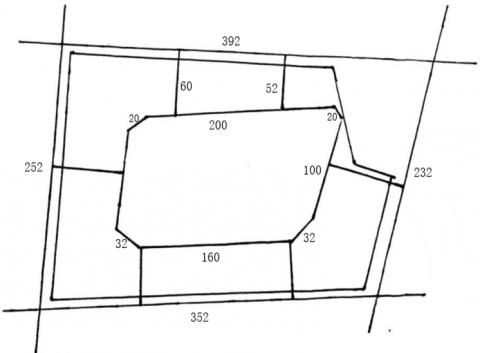Figure 2. Road network map of Jiuzhou Xinshijie Huayuan If a residential community is closed, none of the routes will pass through the residential community, so the traffic flow from outside the residential community were not allocated to the basic routes inside it. After the simulation program was run, with the default speed of the vehicles set to 40km/h, it could be clearly seen that the traffic volumes at intersections increased significantly, as shown in Figure 3. Subsequently, observation points were set on each trunk road segment around the residential community to monitor and sample the traffic flow during the one-hour (7:30-8:30 a.m.) simulation, and the obtained data were analyzed and calculated. The calculation results of the proposed evaluation indicators mentioned in Section 2 are shown in Table 6. Then, the residential community was opened. After simulation, it was found that, under the same conditions, the internal roads of the residential community shared part of the traffic outside the residential community after the residential community was opened, and that the traffic flow on the road segments improved significantly – the traffic volume per unit time was obviously improved, as shown in Figure 4. Then, observation points were set on the trunk road segments at the same locations as above to monitor and sample the traffic flow during the one-hour simulation. The obtained data were analyzed and calculated, with the calculation results of each evaluation indicator shown in Table 7. Through comparison of the changes in various evaluation indicators, it can be seen that the opening of the Type C residential community obviously improved the surrounding road traffic conditions. The differences between various indicators before and after opening are shown in Figure 5 and Figure 6. Table 6. Statistics of the evaluation indicators for closed Jiuzhou Xinshijie Huayuan  Road segment West Laodong Road West Jinling Road West Qingliang Road Xinyi Road Traffic density (pcu/km) 684.56 1016.58 597.16 1118.40 Road congestion rate (%) 43.48 66.79 51.25 69.14 Road accessibility (%) 52.91 55.29 50.28 51.63 Traffic delay time (s) 62.55 Number of intersection conflicts 42.43 Table 7. Statistics of the evaluation indicators for simulated open Jiuzhou Xinshijie Huayuan  Road segment West Laodong Road West Jinling Road West Qingliang Road Xinyi Road Traffic density (pcu/km) 537.26 819.34 446.59 836.99 Road congestion rate (%) 39.25 64.26 46.46 62.71 Road accessibility (%) 56.34 58.29 61.03 54.33 Traffic delay time (s) 41.10 Number of intersection conflicts 32.98 ## 3.png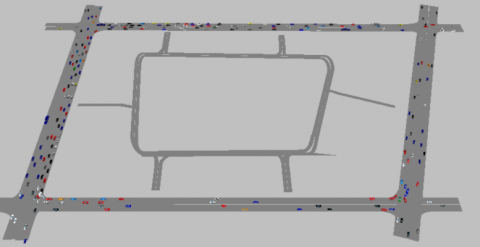Figure 3. Surrounding traffic condition diagram of Jiuzhou Xinshijie Huayuan ## 4.png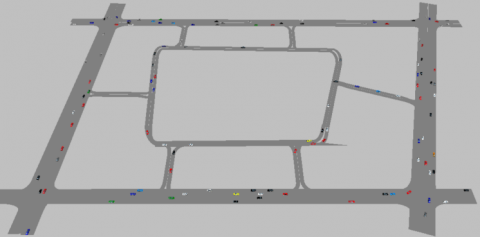Figure 4. Effect picture of Jiuzhou Xinshijie Huayuan after being opened ## 5.png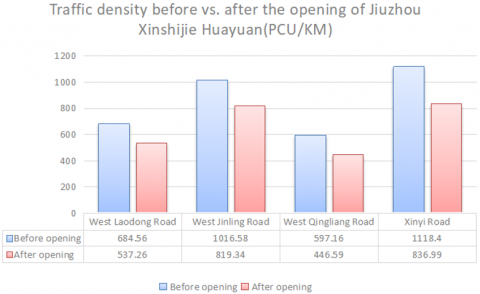Figure 5. Comparison of traffic flow ## 6.png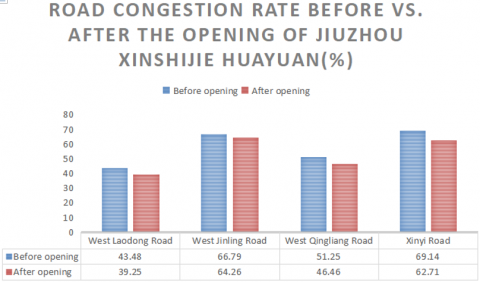Figure 6. Comparison of road congestion rates 3.3 Comparison model for open residential communities based on the grey relational algorithm For the four types of open residential communities, this section uses the grey relational algorithm and combines quantitative and qualitative methods to make the original complex multi-factor comparison problem clearer and simpler and more convenient for calculation, and also to exclude the subjective arbitrariness of the observers to some extent. In this way, the effect relational grade sequence after the opening of the residential communities can be obtained objectively. In short, the grey relational grade analysis means that during the development of a system, if two factors change in the same way, that is, the degree of synchronous change is high, then the two factors can be considered as more related; otherwise, they are less related. Therefore, the grey relational analysis provides a way to quantitatively measure the development and change of a system, which is quite applicable to dynamic process analysis. 3.3.1 Overview of the grey relational algorithm Grey relational analysis refers to the analysis of development trends based on the closeness of the sequence curve shapes of factors, and then provides some suggestions for decision makers . The grey system theory puts forward the idea of performing grey relational analysis on each subsystem. The intention is to find out the numerical relationship between the subsystems (or factors) in the system by a certain method. 3.3.2 Specific steps of the grey relational algorithm (1) Determine the comparison objects (evaluation target) and the reference sequence (evaluation criteria) Suppose there are m evaluation objects and n evaluation indicators, the reference sequence is x0={x0(k)|k=1, 2, ..., n}, and the comparison sequence is xi={xi(k)|k=1, 2, ..., n}, i=1, 2, ..., m. (2) Determine the weight of each indicator value By such methods as AHP, the weights corresponding to each indicator$\varpi=\left[\varpi_{1}, \varpi_{2}, \dots, \varpi_{n}\right]$can be obtained, where, ϖk, k=1, 2, ..., n, is the weight corresponding to the k-th evaluation indicator. (3) Calculate the grey relational coefficient$\xi_{i}(k)=\frac{\min _{s} \min _{t}\left|x_{0}(t)-x_{s}(t)\right|+\rho \max _{s} \tan \left|x_{0}(t)-x_{s}(t)\right|}{\left|x_{0}(k)-x_{i}(k)\right|+\rho \max _{s} \max _{t}\left|x_{0}(t)-x_{s}(t)\right|}$is the relational coefficient of the comparison sequence xi to the reference sequence x0 on the k-th indicator, where$\rho \in[0,1]$is the resolution coefficient . In the formula,$\min _{s} \min _{t}\left|x_{0}(t)-x_{s}(t)\right|$and$\max _{s} \max _{t}\left|x_{0}(t)-x_{s}(t)\right|$are the two-stage minimum difference and the two-stage maximum difference, respectively. Generally speaking, the larger the resolution coefficient ρ, the greater the resolution; vice versa. (4) Calculate the grey weighted relation The calculation formula of the grey weighted relational grade is$r_{i}=\sum_{k=1}^{n} \varpi_{i} \xi_{i}(k)$, where ri is the grey weighted relational grade of the i-th evaluation object to the ideal object. (5) Perform evaluation analysis According to the magnitude of the grey weighted relational grade, the evaluation objects are sorted to form the relational sequence of evaluation objects. The greater the relational grade, the better the evaluation result. 3.3.3 Application of the grey relational algorithm in this study Traffic density, traffic delay time and number of intersection conflicts are cost-based indicators while road congestion rate and road accessibility are efficiency-based indicators, and each indicator has a different dimension. In this paper, the changes in the above indicators are normalized, and the mean value method is adopted, that is, the value of each sequence is compared with the mean value of the entire line sequence. After normalization, the data of the comparison sequence and the reference sequence are shown in Table 8. The maximum value of the changes in each indicator is taken as the value of the virtual optimal residential community. Let ρ=0.5, and calculate$\xi_{i}(k)\$ and ri. The specific values of ri are listed in Table 9.

As shown in Table 9, the grey relational grade sequence is r3>r1>r2> r4; in other words, the surrounding road traffic is significantly improved for Type A and C residential communities after they are opened, followed by that of Type B residential communities, and that of Type D is not obviously improved.

Table 8. Values of the comparison sequence and the reference sequence

 Evaluation indicator 4 types of open residential communities Virtual optimal residential community Type A Type B Type C Type D Traffic density (pcu/km) 1.0806 0.8170 2.0012 0.1017 2.0012 Road congestion rate (%) 1.4047 1.3721 1.1767 0.0465 1.4047 Road accessibility (%) 0.8960 1.4109 1.4851 0.2030 1.4851 Traffic delay time (s) 0.0034 0.2343 3.7500 0.0087 3.7500 Number of intersection conflicts 0.7609 0.7885 2.1724 0.2805 2.1724

Table 9. Value of the grey relational grade of each residential community type
 Type of residential community Type A Type B Type C Type D Grey weighted relational grade ri 0.6130 0.4560 0.6527 0.0036
4. Conclusions

Based on the established vehicle traffic model, this paper employs the VISSIM traffic simulation technology to perform simulation test on a residential community, which directly shows the effect of traffic improvement after the residential community is opened. Through data analysis, the following conclusions are drawn: for residential communities with a large area and large traffic volume and those with a small area and large traffic volume, the surrounding road traffic is significantly reduced and the traffic pressure is greatly alleviated after the opening; the effect comes second for those with a large area and small traffic volume; and for those with a small area and small traffic volume, surrounding traffic sees no improvement. However, in this study, the impact of pedestrians on road traffic is deliberately ignored in the simulation. In fact, after a residential community is opened, pedestrians and vehicles will inevitably move in parallel in the residential community. Regarding this problem, a table of interference coefficients showing how pedestrians affect road traffic can be created and incorporated into the model. In addition, this study is also based on the assumption that there are no traffic lights at the intersections of the roads within the residential community. This assumption has some basis but cannot be applied to all circumstances. If the traffic in the residential community is too heavy, it will be necessary to set traffic lights. Traffic lights are a very important element in the construction of road networks, and their settings are also related to the types of intersections. Therefore, future research may take the types of intersections into account and add traffic lights in the scenarios to better simulate the actual situation after the opening of residential communities.

References

 Guegan, D.P., Martin, P.T., Cottrell, W.D. (2000). Prioritizing traffic-calming projects using the analytic hierarchy process. Transportation Research Record, 1708(1): 61-67. https://doi.org/10.3141/1708-07

 Gong, H.J., Li, B.C. (2006). Application of fuzzy comprehensive evaluation method in road transportation enterprises safety evaluation. Journal of Safety and Environment, 6(3): 54-57. https://doi.org/10.3969/j.issn.1009-6094.2006.03.017

 Chang, Z.Y., Sa, S.L., Fan, P.F. (2004). The first aid of urban accident based on GIS using AHP. Journal of Wuhan University of Technology, 28(2): 255-257. https://doi.org/10.3963/j.issn.2095-3844.2004.02.028

 Wang, E.M., Liu, X.J. (2007). Application of analytic hierarchy process and means of fuzzy comprehensive evaluating in choosing optimal plan of design about energy efficient residential buildings. Sichuan Building Science, 33(2): 146-149. https://doi.org/10.3969/j.issn.1008-1933.2007.02.040

 Wang, L.H., Zhang, Y. (2011). Application of the AHP and entropy weight method in evaluation on suitability of the urban construction land in Tianjin. Geological Survey and Research, 34(4): 305-312. https://doi.org/10.3969/j.issn.1672-4135.2011.04.009

 Yu, J.F., Li, W., Gong, X.L. (2013). Study on the status evaluation of urban road intersections traffic congestion base on AHP-TOPSIS modal. Procedia - Social and Behavioral Sciences, 96: 609-616. https://doi.org/10.1016/j.sbspro.2013.08.071

 Nosal, K., Soleck, K. (2014). Application of AHP method for multi-criteria evaluation of variants of the integration of urban public transport. Transportation Research Procedia, 3: 269-278. https://doi.org/10.1016/j.trpro.2014.10.006

 Żak, J., Kruszyński, M. (2015). Application of AHP and ELECTRE III/IV methods to multiple level, multiple criteria evaluation of urban transportation projects. Transportation Research Procedia, 10: 820-830. https://doi.org/10.1016/j.trpro.2015.09.035

 Barić, D., Pilko, H., Strujić, J. (2016). An analytic hierarchy process model to evaluate road section design. Transport, 31(3): 312-321. https://doi.org/10.3846/16484142.2016.1157830

 Li, H.M., Ni, F.J., Dong, Q., Zhui, Y.Q. (2018). Application of analytic hierarchy process in network level pavement maintenance decision-making. International Journal of Pavement Research and Technology, 11(4): 345-354. https://doi.org/10.1016/j.ijprt.2017.09.015

 Mei, X.B., Wang, F.G., Cao, J.F. (2000). The application of fuzzy comprehensive evaluation on water quality and discussion. World Geology, 19(2): 172-177.

 Yu, K.L., Yang, Y.S., Zhang, C.P. (2007). Application of fuzzy comprehensive evaluation method in identifying water source of water-rush in underground shaft. Metal Mine, (3): 47-50. https://doi.org/10.3321/j.issn:1001-1250.2007.03.013

 Wang, X., Huo, D.L. (2008). Application of fuzzy comprehensive evaluation in coal safety assessment. China Mining Magazine, 17(5): 75-78. https://doi.org/10.3969/j.issn.1004-4051.2008.05.022

 Shao, H.Y., Liu, Q., Li, X.L. (2006). Application of AHP in designing of wastewater treatment plant. Environmental Science & Technology, 29(2): 98-100. https://doi.org/10.3969/j.issn.1003-6504.2006.02.040

 Yang, H.Z., Kang, F.J., Li, J. (2002). The application of AHP in integrative process of assessing the credibility of underwater vehicle system simulation. Acta Simulata Systematica Sinica, 14(10): 1299-1301. https://doi.org/10.3969/j.issn.1004-731X.2002.10.010

 Mogridge, M.J. (1997). The self-defeating nature of urban road capacity policy: A review of theories, disputes and available evidence. Transport Policy, 4(1): 5-23. https://doi.org/10.1016/S0967-070X(96)00030-3

 Rossi, G., Lombardi, M., Mascio, P.D. (2018). Consistency and stability of risk indicators: The case of road infrastructures. International Journal of Safety and Security Engineering, 8(1): 39-47. https://doi.org/10.2495/SAFE-V8-N1-39-47

 Yang, X.G., Lao, Y.T., Yun, M.P. (2007). Application of different pedestrian cross pattern to no-signal controlled segment. Journal of Tongji University, 35(11): 1466-1469. https://doi.org/10.3321/j.issn:0253-374X.2007.11.005

 Siregar, M.L., Agah, H.R., Hidayatullah, F. (2018). Near-miss accident analysis for traffic safety improvement at a ‘channelized’ junction with u-turn. International Journal of Safety and Security Engineering, 8(1): 31-38. https://doi.org/10.2495/SAFE-V8-N1-31-38

 Ziegelmeyer, A., Koessler, F., My, K.B., Denant-Boèmont, L. (2008). Road traffic congestion and public information: An experimental investigation. Journal of Transport Economics and Policy, 42(1): 43-82.

 Olsson, J. (2009). Improved road accessibility and indirect development effects: Evidence from rural Philippines. Journal of Transport Geography, 17(6): 476-483.

 Du, H.B., Mulley, C. (2006). Relationship between transport accessibility and land value: Local model approach with geographically weighted regression. Transportation Research Record, 1977(1): 197-205. https://doi.org/10.1177/0361198106197700123

 Lu, T.C., Kang, K. (2009). The application of entropy method and AHP in weight determining. Computer Programming Skills & Maintenance, (22): 19-22. https://doi.org/10.3969/j.issn.1006-4052.2009.22.009

 Feng, S., Li, L.D. (1999). Decision support for fuzzy comprehensive evaluation of urban development. Fuzzy Sets and Systems, 105(1): 1-12. https://doi.org/10.1016/S0165-0114(97)00229-7

 Wang, Y., Yang, W.F., Li, M., Liu, X. (2012). Risk assessment of floor water inrush in coal mines based on secondary fuzzy comprehensive evaluation. International Journal of Rock Mechanics and Mining Sciences, 52: 50-55. https://doi.org/10.1016/j.ijrmms.2012.03.006

 Kuo, Y., Yang, T., Huang, G.W. (2008). The use of grey relational analysis in solving multiple attribute decision-making problems. Computers & Industrial Engineering, 55(1): 80-93. https://doi.org/10.1016/j.cie.2007.12.002

 Singh, P.N., Raghukandan, K., Pai, B.C. (2004). Optimization by grey relational analysis of EDM parameters on machining Al-10%SiCP composites. Journal of Materials Processing Technology, 155-156: 1658-1661. https://doi.org/10.1016/j.jmatprotec.2004.04.322